Share

RD Sharma solutions for Class 9 Mathematics chapter 13 - Quadrilaterals

Mathematics for Class 9 by R D Sharma (2018-19 Session)

RD Sharma Mathematics Class 9 by R D Sharma (2018-19 Session)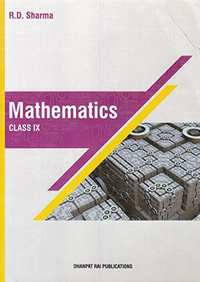Ex. 13.10Ex. 13.20Ex. 13.30Ex. 13.40Others

Chapter 13: Quadrilaterals Exercise 13.10 solutions [Page 4]

Ex. 13.10 | Q 1 | Page 4

Three angles of a quadrilateral are respectively equal to 110°, 50° and 40°. Find its fourth angle

Ex. 13.10 | Q 2 | Page 4

In a quadrilateral ABCD, the angles A, B, C and D are in the ratio 1 : 2 : 4 : 5. Find the measure of each angles of the quadrilateral

Ex. 13.10 | Q 3 | Page 4

The angles of a quadrilateral are in the ratio 3 : 5 : 9 : 13 Find all the angles of the quadrilateral.

Ex. 13.10 | Q 4 | Page 4

In a quadrilateral ABCD, CO and DO are the bisectors of ∠C and ∠D respectively. Prove that

∠COD = 1/2 (∠A+ ∠B).

Chapter 13: Quadrilaterals Exercise 13.20 solutions [Pages 19 - 20]

Ex. 13.20 | Q 1 | Page 19

Two opposite angles of a parallelogram are (3x – 2)° and (50 – x)°. Find the measure of each angle of the parallelogram .

Ex. 13.20 | Q 2 | Page 20

If an angle of a parallelogram is two-third of its adjacent angle, find the angles of the parallelogram .

Ex. 13.20 | Q 3 | Page 20

Find the measure of all the angles of a parallelogram, if one angle is 24° less than twice the smallest angle

Ex. 13.20 | Q 4 | Page 20

The perimeter of a parallelogram is 22 cm .  If the longer side measures 6.5 cm what is the measure of the shorter side?

Ex. 13.20 | Q 5 | Page 20

In a parallelogram ABCD, ∠D = 135°, determine the measures of ∠A and ∠B

Ex. 13.20 | Q 6 | Page 20

ABCD is a parallelogram in which ∠A = 70°. Compute ∠B, ∠C and ∠D .

Ex. 13.20 | Q 7 | Page 20

In Fig. below, ABCD is a parallelogram in which ∠DAB = 75° and ∠DBC = 60°. Compute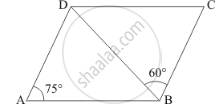Ex. 13.20 | Q 8.1 | Page 20

The following statement are true  and false .

In a parallelogram, the diagonals are equal

Ex. 13.20 | Q 8.2 | Page 20

The following statement are true  and false.

In a parallelogram, the diagonals bisect each other.

Ex. 13.20 | Q 8.3 | Page 20

The following statement are true  and false .

In a parallelogram, the diagonals intersect each other at right angles .

Ex. 13.20 | Q 8.4 | Page 20

The following statement are true  and false .

In any quadrilateral, if a pair of opposite sides is equal, it is a parallelogram.

Ex. 13.20 | Q 8.5 | Page 20

The following statement are true  and false .

If all the angles of a quadrilateral are equal, it is a parallelogram .

Ex. 13.20 | Q 8.6 | Page 20

The following statement are true  and false .

If three sides of a quadrilateral are equal, it is a parallelogram .

Ex. 13.20 | Q 8.7 | Page 20

The following statement are true  and false .

If three angles of a quadrilateral are equal, it is a parallelogram .

Ex. 13.20 | Q 8.8 | Page 20

The following statement are true  and false .

If all the sides of a quadrilateral are equal it is a parallelogram.

Ex. 13.20 | Q 9 | Page 20

In Fig., below, ABCD is a parallelogram in which ∠A = 60°. If the bisectors of ∠A and ∠B meet at P, prove that AD = DP, PC = BC and DC = 2AD.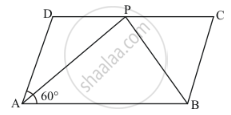Ex. 13.20 | Q 9 | Page 20

In Fig., below, ABCD is a parallelogram in which ∠A = 60°. If the bisectors of ∠A and ∠B meet at P, prove that AD = DP, PC = BC and DC = 2AD.Ex. 13.20 | Q 10 | Page 20

In below fig. ABCD is a parallelogram and E is the mid-point of side B If DE and AB when produced meet at F, prove that AF = 2AB.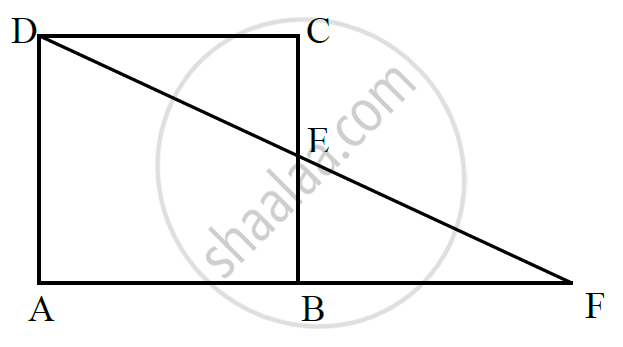Chapter 13: Quadrilaterals Exercise 13.30 solutions [Pages 42 - 43]

Ex. 13.30 | Q 1 | Page 42

In a parallelogram ABCD, determine the sum of angles ∠C and ∠D .

Ex. 13.30 | Q 1 | Page 42

In a parallelogram ABCD, determine the sum of angles ∠C and ∠D .

Ex. 13.30 | Q 2 | Page 42

In a parallelogram ABCD, if ∠B = 135°, determine the measures of its other angles .

Ex. 13.30 | Q 2 | Page 42

In a parallelogram ABCD, if ∠B = 135°, determine the measures of its other angles .

Ex. 13.30 | Q 3 | Page 42

ABCD is a square. AC and BD intersect at O. State the measure of ∠AOB.

Ex. 13.30 | Q 3 | Page 42

ABCD is a square. AC and BD intersect at O. State the measure of ∠AOB.

Ex. 13.30 | Q 4 | Page 42

ABCD is a rectangle with ∠ABD = 40°. Determine ∠DBC .

Ex. 13.30 | Q 5 | Page 42

The sides AB and CD of a parallelogram ABCD are bisected at E and F. Prove that EBFD is a parallelogram.

Ex. 13.30 | Q 5 | Page 42

The sides AB and CD of a parallelogram ABCD are bisected at E and F. Prove that EBFD is a parallelogram.

Ex. 13.30 | Q 6 | Page 42

P and Q are the points of trisection of the diagonal BD of a parallelogram AB Prove that CQ is parallel to AP. Prove also that AC bisects PQ.

Ex. 13.30 | Q 6 | Page 42

P and Q are the points of trisection of the diagonal BD of a parallelogram AB Prove that CQ is parallel to AP. Prove also that AC bisects PQ.

Ex. 13.30 | Q 7 | Page 43

ABCD is a square E, F, G and H are points on AB, BC, CD and DA respectively, such that AE = BF = CG = DH. Prove that EFGH is a square.

Ex. 13.30 | Q 8 | Page 43

ABCD is a rhombus, EABF is a straight line such that EA = AB = BF. Prove that ED and FC when produced meet at right angles

Ex. 13.30 | Q 9 | Page 43

ABCD is a parallelogram, AD is produced to E so that DE = DC and EC produced meets AB produced in F. Prove that BF = BC.

Chapter 13: Quadrilaterals Exercise 13.40 solutions [Pages 62 - 65]

Ex. 13.40 | Q 1 | Page 62

In a ∆ABC, D, E and F are, respectively, the mid-points of BC, CA and AB. If the lengths of side AB, BC and CA are 7 cm, 8 cm and 9 cm, respectively, find the perimeter of ∆DEF.

Ex. 13.40 | Q 2 | Page 62

In a triangle ∠ABC, ∠A = 50°, ∠B = 60° and ∠C = 70°. Find the measures of the angles of

the triangle formed by joining the mid-points of the sides of this triangle.

Ex. 13.40 | Q 3 | Page 63

In a triangle, P, Q and R are the mid-points of sides BC, CA and AB respectively. If AC =
21 cm, BC = 29 cm and AB = 30 cm, find the perimeter of the quadrilateral ARPQ.

Ex. 13.40 | Q 4 | Page 63

In a ΔABC median AD is produced to X such that AD = DX. Prove that ABXC is a
parallelogram.

Ex. 13.40 | Q 4 | Page 63

In a ΔABC median AD is produced to X such that AD = DX. Prove that ABXC is a
parallelogram.

Ex. 13.40 | Q 5 | Page 63

In a ΔABC, E and F are the mid-points of AC and AB respectively. The altitude AP to BC
intersects FE at Q. Prove that AQ = QP.

Ex. 13.40 | Q 6 | Page 63

In a ΔABC, BM and CN are perpendiculars from B and C respectively on any line passing
through A. If L is the mid-point of BC, prove that ML = NL.

Ex. 13.40 | Q 7 | Page 63

In Fig. below, triangle ABC is right-angled at B. Given that AB = 9 cm, AC = 15 cm and D,
E are the mid-points of the sides AB and AC respectively, calculate
(i) The length of BC (ii) The area of ΔADE.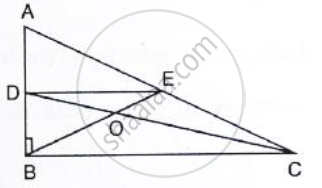Ex. 13.40 | Q 8 | Page 63

In Fig. below, M, N and P are the mid-points of AB, AC and BC respectively. If MN = 3 cm, NP = 3.5 cm and MP = 2.5 cm, calculate BC, AB and AC.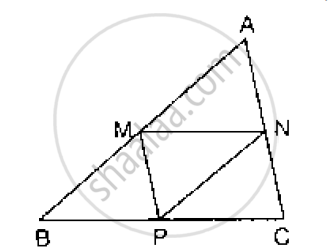Ex. 13.40 | Q 9 | Page 63

In Fig. below, AB = AC and CP || BA and AP is the bisector of exterior ∠CAD of ΔABC.
Prove that (i) ∠PAC = ∠BCA (ii) ABCP is a parallelogram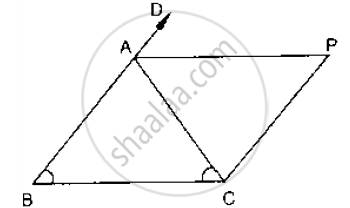Ex. 13.40 | Q 9 | Page 63

In Fig. below, AB = AC and CP || BA and AP is the bisector of exterior ∠CAD of ΔABC.
Prove that (i) ∠PAC = ∠BCA (ii) ABCP is a parallelogramEx. 13.40 | Q 10 | Page 63

ABCD is a kite having AB = AD and BC = CD. Prove that the figure formed by joining the
mid-points of the sides, in order, is a rectangle.

Ex. 13.40 | Q 11 | Page 64

Let Abc Be an Isosceles Triangle in Which Ab = Ac. If D, E, F Be the Mid-points of the Sides Bc, Ca and a B Respectively, Show that the Segment Ad and Ef Bisect Each Other at Right Angles.

Ex. 13.40 | Q 12 | Page 64

Show that the line segments joining the mid-points of the opposite sides of a quadrilateral
bisect each other.

Ex. 13.40 | Q 13.1 | Page 64

Fill in the blank to make the following statement correct

The triangle formed by joining the mid-points of the sides of an isosceles triangle is

Ex. 13.40 | Q 13.2 | Page 64

Fill in the blank to make the following statement correct:

The triangle formed by joining the mid-points of the sides of a right triangle is

Ex. 13.40 | Q 13.3 | Page 64

Fill in the blank to make the following statement correct:

The figure formed by joining the mid-points of consecutive sides of a quadrilateral is

Ex. 13.40 | Q 14 | Page 64

ABC is a triangle and through A, B, C lines are drawn parallel to BC, CA and AB respectively
intersecting at P, Q and R. Prove that the perimeter of ΔPQR is double the perimeter of
ΔABC

Ex. 13.40 | Q 15 | Page 64

In Fig. below, BE ⊥ AC. AD is any line from A to BC intersecting BE in H. P, Q and R are
respectively the mid-points of AH, AB and BC. Prove that ∠PQR = 90°.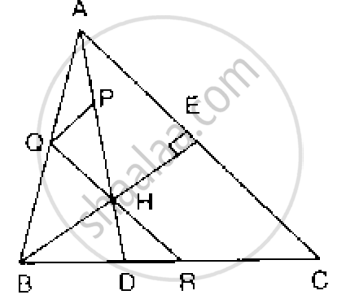Ex. 13.40 | Q 16 | Page 64

ABC is a triang D is a point on AB such that AD = 1/4 AB and E is a point on AC such that AE = 1/4 AC. Prove that DE = 1/4 BC.

Ex. 13.40 | Q 17 | Page 64

In below Fig, ABCD is a parallelogram in which P is the mid-point of DC and Q is a point on AC such that CQ = 1/4 AC. If PQ produced meets BC at R, prove that R is a mid-point of BC.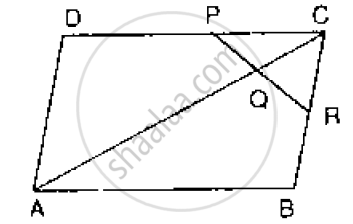Ex. 13.40 | Q 18 | Page 64

In the below Fig, ABCD and PQRC are rectangles and Q is the mid-point of Prove thaT

i) DP = PC (ii) PR = 1/2 AC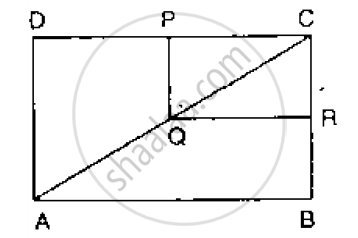Ex. 13.40 | Q 19 | Page 65

ABCD is a parallelogram, E and F are the mid-points of AB and CD respectively. GH is any line intersecting AD, EF and BC at G, P and H respectively. Prove that GP = PH

Ex. 13.40 | Q 20 | Page 65

BM and CN are perpendiculars to a line passing through the vertex A of a triangle ABC. If
L is the mid-point of BC, prove that LM = LN.

Chapter 13: Quadrilaterals solutions [Pages 68 - 70]

Q 1 | Page 68

In a parallelogram ABCD, write the sum of angles A and B.

Q 1 | Page 68

In a parallelogram ABCD, write the sum of angles A and B.

Q 2 | Page 68

In a parallelogram ABCD, if ∠D = 115°, then write the measure of ∠A.

Q 2 | Page 68

In a parallelogram ABCD, if ∠D = 115°, then write the measure of ∠A.

Q 3 | Page 68

PQRS is a square such that PR and SQ intersect at O. State the measure of ∠POQ.

Q 4 | Page 68

If PQRS is a square, then write the measure of ∠SRP.

Q 5 | Page 68

If ABCD is a rhombus with ∠ABC = 56°, find the measure of ∠ACD.

Q 6 | Page 68

The perimeter of a parallelogram is 22 cm. If the longer side measures 6.5 cm, what is the measure of shorter side?

Q 7 | Page 68

If the angles of a quadrilateral are in the ratio 3 : 5 : 9 : 13, then find the measure of the smallest angle.

Q 8 | Page 68

In a parallelogram ABCD, if ∠A = (3x − 20)°, ∠B = (y + 15)°, ∠C = (x + 40)°, then find the values of xand y.

Q 8 | Page 68

In a parallelogram ABCD, if ∠A = (3x − 20)°, ∠B = (y + 15)°, ∠C = (x + 40)°, then find the values of xand y.

Q 9 | Page 69

If measures opposite angles of a parallelogram are (60 − x)° and (3x − 4)°, then find the measures of angles of the parallelogram.

Q 10 | Page 69

In a parallelogram ABCD, the bisector of ∠A also bisects BC at X. Find AB : AD.

Q 10 | Page 69

In a parallelogram ABCD, the bisector of ∠A also bisects BC at X. Find AB : AD.

Q 11 | Page 69

In the given figure, PQRS is an isosceles trapezium. Find x and y.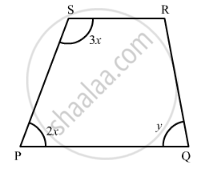Q 12 | Page 69

In the given figure, ABCD is a trapezium. Find the values of x and y.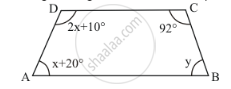Q 13 | Page 69

In the given figure, ABCD and AEFG are two parallelograms. If ∠C = 58°, find ∠F.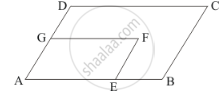Q 14.1 | Page 69

Complete the following statement by means of one of those given in brackets against each:

If one pair of opposite sides are equal and parallel, then the figure is ........................

• parallelogram

• rectangle

• trapezium

Q 14.2 | Page 69

Complete the following statement by means of one of those given in brackets against each:

If in a quadrilateral only one pair of opposite sides are parallel, the quadrilateral is ................

• square

• rectangle

•  trapezium

Q 14.3 | Page 69

Complete the following statement by means of one of those given in brackets against each:

A line drawn from the mid-point of one side of a triangle .............. another side intersects the third side at its mid-point.

• perpendicular to parallel to

• to meet

Q 14.4 | Page 69

Complete the following statement by means of one of those given in brackets against each:

If one angle of a parallelogram is a right angle, then it is necessarily a .................

• rectangle

• square

• rhombus

Q 14.5 | Page 69

Complete the following statement by means of one of those given in brackets against each:

Consecutive angles of a parallelogram are ...................

• supplementary

• complementary

Q 14.6 | Page 69

Complete the following statement by means of one of those given in brackets against each:

If both pairs of opposite sides of a quadrilateral are equal, then it is necessarily a ...............

• rectangle

• parallelogram

• rhombus

Q 14.7 | Page 69

Complete the following statement by means of one of those given in brackets against each:

If opposite angles of a quadrilateral are equal, then it is necessarily a ....................

• parallelogram

•  rhombus

•  rectangle

Q 14.8 | Page 69

Complete the following statement by means of one of those given in brackets against each:

f consecutive sides of a parallelogram are equal, then it is necessarily a ..................

• kite

• rhombus

• square

Q 15 | Page 69

In a quadrilateral ABCD, bisectors of angles A and B intersect at O such that ∠AOB = 75°, then write the value of ∠C + ∠D.

Q 16 | Page 69

The diagonals of a rectangle ABCD meet at O, If ∠BOC = 44°, find ∠OAD.

Q 17 | Page 70

If ABCD is a rectangle with ∠BAC = 32°, find the measure of ∠DBC.

Q 18 | Page 70

If the bisectors of two adjacent angles A and B of a quadrilateral ABCD intersect at a point O such that ∠C + ∠D = k ∠AOB, then find the value of k.

Q 19 | Page 70

In the given figure, PQRS is a rhombus in which the diagonal PR is produced to T. If ∠SRT = 152°, find x, y and z.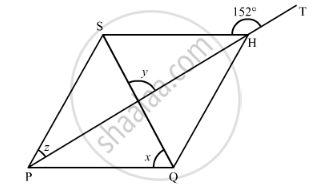Q 20 | Page 70

In the given figure, ABCD is a rectangle in which diagonal AC is produced to E. If ∠ECD = 146°, find ∠AOB.

Chapter 13: Quadrilaterals solutions [Pages 70 - 73]

Q 1 | Page 70

Mark the correct alternative in each of the following:
The opposite sides of a quadrilateral have

•  no common point

•  one common point

•  two common points

• infinitely many common points

Q 2 | Page 70

The consecutive sides of a quadrilateral have

•  no common point

• one common point

•  two common points

•  infinitely many common points

Q 3.1 | Page 71

PQRS is a quadrilateral, PR and QS intersect each other at O. In which of the following case, PQRS is a parallelogram?

∠P = 100°, ∠Q = 80°, ∠R = 95°

Q 3.1 | Page 71

PQRS is a quadrilateral, PR and QS intersect each other at O. In which of the following case, PQRS is a parallelogram?

∠P = 100°, ∠Q = 80°, ∠R = 95°

Q 3.2 | Page 71

PQRS is a quadrilateral, PR and QS intersect each other at O. In which of the following case, PQRS is a parallelogram?

∠P =85°, ∠Q = 85°, ∠R = 95°

Q 3.3 | Page 71

PQRS is a quadrilateral, PR and QS intersect each other at O. In which of the following case, PQRS is a parallelogram?

PQ = 7 cm, QR = 7 cm, RS = 8 cm, SP = 8 cm

Q 3.4 | Page 71

PQRS is a quadrilateral, PR and QS intersect each other at O. In which of the following case, PQRS is a parallelogram?

OP = 6.5 cm, OQ = 6.5 cm, OR = 5.2 cm, OS = 5.2 cm

Q 4 | Page 71

Which  of the following quadrilateral is not a rhombus?

• All four sides are equal

• Diagonals bisect each other

• Diagonals bisect opposite angles

• One angle between the diagonals is 60°

Q 5 | Page 71

Diagonals necessarily bisect opposite angles in a

• rectangle

• parallelogram

• isosceles trapezium

• square

Q 6 | Page 71

The two diagonals are equal in a

• parallelogram

•  rhombus

•  rectangle

• trapezium

Q 7 | Page 71

We get a rhombus by joining the mid-points of the sides of a

• parallelogram

• rhombus

• rectangle

• triangle

Q 7 | Page 71

We get a rhombus by joining the mid-points of the sides of a

• parallelogram

• rhombus

• rectangle

• triangle

Q 8 | Page 71

The bisectors of any two adjacent  angles of a parallelogram intersect at

• 30°

• 45°

• 60°

• 90°

Q 9 | Page 71

The bisectors of the angle of a parallelogram enclose a

• parallelogram

•  rhombus

•  rectangle

• square

Q 10 | Page 71

The figure formed by joining the mid-points of the adjacent sides of a quadrilateral is a

• parallelogram

• rectangle

• square

• rhombus

Q 11 | Page 71

The figure formed by joining the mid-points of the adjacent sides of a rectangle is a

• square

• rhombus

• trapezium

• none of these

Q 12 | Page 71

The figure formed by joining the mid-points of the adjacent sides of a rhombus is a

•  square

• rectangle

• trapezium

• none of these

Q 12 | Page 71

The figure formed by joining the mid-points of the adjacent sides of a rhombus is a

•  square

• rectangle

• trapezium

• none of these

Q 13 | Page 71

The figure formed by joining the mid-points of the adjacent sides of a square is a

• rhombus

• square

• rectangle

• parallelogram

Q 14 | Page 71

The figure formed by joining the mid-points of the adjacent sides of a parallelogram is a

• rectangle

• parallelogram

• rhombus

• square

Q 14 | Page 71

The figure formed by joining the mid-points of the adjacent sides of a parallelogram is a

• rectangle

• parallelogram

• rhombus

• square

Q 15 | Page 71

If one angle of a parallelogram is 24° less than twice the smallest angle, then the measure of the largest angle of the parallelogram is

• 176°

• 68°

•  112°

• 102°

Q 16 | Page 71

In a parallelogram ABCD, if ∠DAB = 75° and ∠DBC = 60°, then ∠BDC =

• 75°

• 60°

•  45°

• 55°

Q 17 | Page 71

ABCD is a parallelogram and E and F are the centroids of triangles ABD and BCDrespectively, then EF =

• AE

• BE

• CE

• DE

Q 18 | Page 72

ABCD is a parallelogram, M is the mid-point of BD and BM bisects ∠B. Then ∠AMB =

• 45°

• 60°

• 90°

•  75°

Q 19 | Page 72

If an angle of a parallelogram is two-third of its adjacent angle, the smallest angle of the parallelogram is

• 108°

• 54°

• 72°

• 81°

Q 20 | Page 72

If the degree measures of the angles of quadrilateral are 4x, 7x, 9x and 10x, what is the sum of the measures of the smallest angle and largest angle?

• 140°

• 150°

• 168°

•  180°

Q 21 | Page 72

If the diagonals of a rhombus are 18 cm and 24 cm respectively, then its side is equal to

• 16 cm

• 15 cm

• 20 cm

• 17 cm

Q 22 | Page 72

ABCD is a parallelogram in which diagonal AC bisects ∠BAD. If ∠BAC = 35°, then ∠ABC =

• 70°

• 110°

• 90°

• 120°

Q 22 | Page 72

ABCD is a parallelogram in which diagonal AC bisects ∠BAD. If ∠BAC = 35°, then ∠ABC =

• 70°

• 110°

• 90°

• 120°

Q 23 | Page 72

In a rhombus ABCD, if ∠ACB = 40°, then ∠ADB =

•  70°

• 45°

• 50°

• 60°

Q 24 | Page 72

In ΔABC, ∠A = 30°, ∠B = 40° and ∠C = 110°. The angles of the triangle formed by joining the mid-points of the sides of this triangle are

• 70°, 70°, 40°

• 60°, 40°, 80°

• 30°, 40°, 110°

• 60°, 70°, 50°

Q 25 | Page 72

The diagonals of a parallelogram ABCD intersect at O. If ∠BOC = 90° and ∠BDC = 50°, then ∠OAB =

• 40°

• 50°

• 10°

• 90°

Q 26 | Page 72

ABCD is a trapezium in which AB || DC. M and N are the mid-points of AD and the respectively. If AB = 12 cm, MN = 14 cm, then CD =

• 10 cm

• 12 cm

• 14 cm

• 16 cm

Q 27 | Page 72

Diagonals of a quadrilateral ABCD bisect each other. If ∠A= 45°, then ∠B =

• 115°

• 120°

• 125°

• 135°

Q 28 | Page 72

P is the mid-point of side BC of a parallelogram ABCD such that ∠BAP = ∠DAP. If AD = 10 cm, then CD =

• 5 cm

• 6 cm

• 8 cm

•  10 cm

Q 28 | Page 72

P is the mid-point of side BC of a parallelogram ABCD such that ∠BAP = ∠DAP. If AD = 10 cm, then CD =

• 5 cm

• 6 cm

• 8 cm

•  10 cm

Q 29 | Page 72

In ΔABC, E is the mid-point of median AD such that BE produced meets AC at F. IF AC = 10.5 cm, then AF =

•  3 cm

• 3.5 cm

• 2.5 cm

•  5 cm

Q 30 | Page 72

ABCD is a parallelogram and E is the mid-point of BC. DE and AB when produced meet at F. Then, AF =

• $\frac{3}{2}AB$

• 2 AB

• 3 AB

• $\frac{5}{4}AB$

Q 31 | Page 73

In a quadrilateral ABCD, ∠A + ∠C is 2 times ∠B + ∠D. If ∠A = 140° and ∠D = 60°, then ∠B=

• 60°

• 80°

• 120°

• 80°

• None of these

Q 31 | Page 73

In a quadrilateral ABCD, ∠A + ∠C is 2 times ∠B + ∠D. If ∠A = 140° and ∠D = 60°, then ∠B=

• 60°

• 80°

• 120°

• 80°

• None of these

Q 32 | Page 73

The diagonals  AC and BD of a rectangle ABCD intersect each other at P. If ∠ABD = 50°, then ∠DPC =

•  70°

• 90°

• 80°

• 100°

Ex. 13.10Ex. 13.20Ex. 13.30Ex. 13.40Others

RD Sharma Mathematics Class 9 by R D Sharma (2018-19 Session)RD Sharma solutions for Class 9 Mathematics chapter 13 - Quadrilaterals

RD Sharma solutions for Class 9 Maths chapter 13 (Quadrilaterals) include all questions with solution and detail explanation. This will clear students doubts about any question and improve application skills while preparing for board exams. The detailed, step-by-step solutions will help you understand the concepts better and clear your confusions, if any. Shaalaa.com has the CBSE Mathematics for Class 9 by R D Sharma (2018-19 Session) solutions in a manner that help students grasp basic concepts better and faster.

Further, we at Shaalaa.com are providing such solutions so that students can prepare for written exams. RD Sharma textbook solutions can be a core help for self-study and acts as a perfect self-help guidance for students.

Concepts covered in Class 9 Mathematics chapter 13 Quadrilaterals are Concept of Quadrilaterals, Angle Sum Property of a Quadrilateral, Types of Quadrilaterals, Another Condition for a Quadrilateral to Be a Parallelogram, The Mid-point Theorem, Theorem : a Diagonal of a Parallelogram Divides It into Two Congruent Triangles, Theorem : a Diagonal of a Parallelogram Divides It into Two Congruent Triangles, In a Parallelogram, Opposite Sides Are Equal. Ab = Cd and Bc = Da, Theorem : If Each Pair of Opposite Sides of a Quadrilateral is Equal, Then It is a Parallelogram., In a Parallelogram, Opposite Angles Are Equal., Theorem: If in a Quadrilateral, Each Pair of Opposite Angles is Equal, Then It is a Parallelogram., The Diagonals of a Parallelogram Bisect Each Other., Theorem : If the Diagonals of a Quadrilateral Bisect Each Other, Then It is a Parallelogram.

Using RD Sharma Class 9 solutions Quadrilaterals exercise by students are an easy way to prepare for the exams, as they involve solutions arranged chapter-wise also page wise. The questions involved in RD Sharma Solutions are important questions that can be asked in the final exam. Maximum students of CBSE Class 9 prefer RD Sharma Textbook Solutions to score more in exam.

Get the free view of chapter 13 Quadrilaterals Class 9 extra questions for Maths and can use Shaalaa.com to keep it handy for your exam preparation

S